login  home  contents  what's new  discussion  bug reports help  links  subscribe  changes  refresh  edit
Description
DifferentialSparseMultivariatePolynomial? implements an ordinary differential polynomial ring by combining a domain belonging to the category DifferentialVariableCategory? with the domain SparseMultivariatePolynomial?.
Author
William Sit
Date Created
19 July 1990
Date Last Updated
13 September 1991

Basic Operations

fricas
)show DifferentialPolynomialCategory
DifferentialPolynomialCategory(R: Ring,S: OrderedSet,V: DifferentialVariableCategory(t#2),E: OrderedAbelianMonoidSup) is a category constructor
Abbreviation for DifferentialPolynomialCategory is DPOLCAT
This constructor is exposed in this frame.
------------------------------- Operations --------------------------------
?*? : (Integer, %) -> %               ?*? : (R, %) -> %
?*? : (%, R) -> %                     ?*? : (%, %) -> %
?*? : (PositiveInteger, %) -> %       ?+? : (%, %) -> %
?-? : (%, %) -> %                     -? : % -> %
?=? : (%, %) -> Boolean               D : (%, (R -> R)) -> %
D : % -> % if R has DIFRING           1 : () -> %
0 : () -> %                           ?^? : (%, PositiveInteger) -> %
annihilate? : (%, %) -> Boolean       antiCommutator : (%, %) -> %
associator : (%, %, %) -> %           coefficient : (%, E) -> R
coefficients : % -> List(R)           coerce : S -> %
coerce : Integer -> %                 coerce : R -> %
coerce : % -> OutputForm              commutator : (%, %) -> %
degree : % -> E                       eval : (%, V, %) -> %
eval : (%, V, R) -> %                 ground : % -> R
ground? : % -> Boolean                hash : % -> SingleInteger
initial : % -> %                      isobaric? : % -> Boolean
latex : % -> String                   leader : % -> V
map : ((R -> R), %) -> %              minimumDegree : % -> E
monomial : (R, E) -> %                monomial? : % -> Boolean
monomials : % -> List(%)              one? : % -> Boolean
opposite? : (%, %) -> Boolean         order : % -> NonNegativeInteger
pomopo! : (%, R, E, %) -> %           recip : % -> Union(%,"failed")
retract : % -> S                      retract : % -> R
sample : () -> %                      separant : % -> %
support : % -> List(E)                variables : % -> List(V)
weight : % -> NonNegativeInteger      zero? : % -> Boolean
?~=? : (%, %) -> Boolean
?*? : (NonNegativeInteger, %) -> %
?*? : (%, Fraction(Integer)) -> % if R has ALGEBRA(FRAC(INT))
?*? : (Fraction(Integer), %) -> % if R has ALGEBRA(FRAC(INT))
?/? : (%, R) -> % if R has FIELD
D : (%, (R -> R), NonNegativeInteger) -> %
D : (%, List(Symbol), List(NonNegativeInteger)) -> % if R has PDRING(SYMBOL)
D : (%, Symbol, NonNegativeInteger) -> % if R has PDRING(SYMBOL)
D : (%, List(Symbol)) -> % if R has PDRING(SYMBOL)
D : (%, Symbol) -> % if R has PDRING(SYMBOL)
D : (%, NonNegativeInteger) -> % if R has DIFRING
D : (%, V) -> % if R has RING
D : (%, List(V)) -> % if R has RING
D : (%, V, NonNegativeInteger) -> % if R has RING
D : (%, List(V), List(NonNegativeInteger)) -> % if R has RING
?^? : (%, NonNegativeInteger) -> %
associates? : (%, %) -> Boolean if R has ENTIRER or R has INTDOM and % has ATVCWC
binomThmExpt : (%, %, NonNegativeInteger) -> % if % has COMRING
characteristic : () -> NonNegativeInteger
charthRoot : % -> Union(%,"failed") if and(has($,CharacteristicNonZero),has(R,PolynomialFactorizationExplicit)) or R has CHARNZ coefficient : (%, List(V), List(NonNegativeInteger)) -> % coefficient : (%, V, NonNegativeInteger) -> % coerce : V -> % if R has SRING coerce : % -> % if R has GCDDOM or R has COMRING and % has ATVCWC or R has INTDOM and % has ATVCWC coerce : Fraction(Integer) -> % if R has RETRACT(FRAC(INT)) or R has ALGEBRA(FRAC(INT)) conditionP : Matrix(%) -> Union(Vector(%),"failed") if and(has($,CharacteristicNonZero),has(R,PolynomialFactorizationExplicit))
construct : List(Record(k: E,c: R)) -> %
constructOrdered : List(Record(k: E,c: R)) -> % if E has COMPAR
content : (%, V) -> % if R has GCDDOM
content : % -> R if R has GCDDOM
convert : % -> InputForm if V has KONVERT(INFORM) and R has KONVERT(INFORM)
convert : % -> Pattern(Integer) if V has KONVERT(PATTERN(INT)) and R has KONVERT(PATTERN(INT)) and R has RING
convert : % -> Pattern(Float) if V has KONVERT(PATTERN(FLOAT)) and R has KONVERT(PATTERN(FLOAT)) and R has RING
degree : (%, S) -> NonNegativeInteger
degree : (%, List(V)) -> List(NonNegativeInteger)
degree : (%, V) -> NonNegativeInteger
differentialVariables : % -> List(S)
differentiate : (%, (R -> R)) -> %
differentiate : (%, (R -> R), NonNegativeInteger) -> %
differentiate : (%, List(Symbol), List(NonNegativeInteger)) -> % if R has PDRING(SYMBOL)
differentiate : (%, Symbol, NonNegativeInteger) -> % if R has PDRING(SYMBOL)
differentiate : (%, List(Symbol)) -> % if R has PDRING(SYMBOL)
differentiate : (%, Symbol) -> % if R has PDRING(SYMBOL)
differentiate : (%, NonNegativeInteger) -> % if R has DIFRING
differentiate : % -> % if R has DIFRING
differentiate : (%, V) -> % if R has RING
differentiate : (%, List(V)) -> % if R has RING
differentiate : (%, V, NonNegativeInteger) -> % if R has RING
differentiate : (%, List(V), List(NonNegativeInteger)) -> % if R has RING
discriminant : (%, V) -> % if R has COMRING
eval : (%, List(S), List(R)) -> % if R has DIFRING
eval : (%, S, R) -> % if R has DIFRING
eval : (%, List(S), List(%)) -> % if R has DIFRING
eval : (%, S, %) -> % if R has DIFRING
eval : (%, List(%), List(%)) -> % if R has SRING
eval : (%, %, %) -> % if R has SRING
eval : (%, Equation(%)) -> % if R has SRING
eval : (%, List(Equation(%))) -> % if R has SRING
eval : (%, List(V), List(%)) -> %
eval : (%, List(V), List(R)) -> %
exquo : (%, %) -> Union(%,"failed") if R has ENTIRER or R has INTDOM and % has ATVCWC
exquo : (%, R) -> Union(%,"failed") if R has ENTIRER
factor : % -> Factored(%) if R has PFECAT
factorPolynomial : SparseUnivariatePolynomial(%) -> Factored(SparseUnivariatePolynomial(%)) if R has PFECAT
factorSquareFreePolynomial : SparseUnivariatePolynomial(%) -> Factored(SparseUnivariatePolynomial(%)) if R has PFECAT
fmecg : (%, E, R, %) -> % if R has RING
gcd : (%, %) -> % if R has GCDDOM
gcd : List(%) -> % if R has GCDDOM
gcdPolynomial : (SparseUnivariatePolynomial(%), SparseUnivariatePolynomial(%)) -> SparseUnivariatePolynomial(%) if R has GCDDOM
hashUpdate! : (HashState, %) -> HashState
isExpt : % -> Union(Record(var: V,exponent: NonNegativeInteger),"failed") if R has SRING
isPlus : % -> Union(List(%),"failed")
isTimes : % -> Union(List(%),"failed") if R has SRING
lcm : (%, %) -> % if R has GCDDOM
lcm : List(%) -> % if R has GCDDOM
lcmCoef : (%, %) -> Record(llcm_res: %,coeff1: %,coeff2: %) if R has GCDDOM
leadingCoefficient : % -> R if E has COMPAR
leadingMonomial : % -> % if E has COMPAR
leadingSupport : % -> E if E has COMPAR
leadingTerm : % -> Record(k: E,c: R) if E has COMPAR
leftPower : (%, NonNegativeInteger) -> %
leftPower : (%, PositiveInteger) -> %
leftRecip : % -> Union(%,"failed")
linearExtend : ((E -> R), %) -> R if R has COMRING
listOfTerms : % -> List(Record(k: E,c: R))
mainVariable : % -> Union(V,"failed")
makeVariable : % -> (NonNegativeInteger -> %) if R has DIFRING
makeVariable : S -> (NonNegativeInteger -> %)
mapExponents : ((E -> E), %) -> %
minimumDegree : (%, List(V)) -> List(NonNegativeInteger)
minimumDegree : (%, V) -> NonNegativeInteger
monicDivide : (%, %, V) -> Record(quotient: %,remainder: %) if R has RING
monomial : (%, List(V), List(NonNegativeInteger)) -> %
monomial : (%, V, NonNegativeInteger) -> %
multivariate : (SparseUnivariatePolynomial(%), V) -> %
multivariate : (SparseUnivariatePolynomial(R), V) -> %
numberOfMonomials : % -> NonNegativeInteger
order : (%, S) -> NonNegativeInteger
patternMatch : (%, Pattern(Integer), PatternMatchResult(Integer,%)) -> PatternMatchResult(Integer,%) if V has PATMAB(INT) and R has PATMAB(INT) and R has RING
patternMatch : (%, Pattern(Float), PatternMatchResult(Float,%)) -> PatternMatchResult(Float,%) if V has PATMAB(FLOAT) and R has PATMAB(FLOAT) and R has RING
prime? : % -> Boolean if R has PFECAT
primitiveMonomials : % -> List(%) if R has SRING
primitivePart : (%, V) -> % if R has GCDDOM
primitivePart : % -> % if R has GCDDOM
reducedSystem : Matrix(%) -> Matrix(R) if R has RING
reducedSystem : (Matrix(%), Vector(%)) -> Record(mat: Matrix(R),vec: Vector(R)) if R has RING
reducedSystem : (Matrix(%), Vector(%)) -> Record(mat: Matrix(Integer),vec: Vector(Integer)) if and(has(R,LinearlyExplicitOver(Integer)),has(R,Ring))
reducedSystem : Matrix(%) -> Matrix(Integer) if and(has(R,LinearlyExplicitOver(Integer)),has(R,Ring))
reductum : % -> % if E has COMPAR
resultant : (%, %, V) -> % if R has COMRING
retract : % -> V if R has SRING
retract : % -> Fraction(Integer) if R has RETRACT(FRAC(INT))
retract : % -> Integer if R has RETRACT(INT)
retractIfCan : % -> Union(S,"failed")
retractIfCan : % -> Union(V,"failed") if R has SRING
retractIfCan : % -> Union(R,"failed")
retractIfCan : % -> Union(Fraction(Integer),"failed") if R has RETRACT(FRAC(INT))
retractIfCan : % -> Union(Integer,"failed") if R has RETRACT(INT)
rightPower : (%, NonNegativeInteger) -> %
rightPower : (%, PositiveInteger) -> %
rightRecip : % -> Union(%,"failed")
smaller? : (%, %) -> Boolean if R has COMPAR
solveLinearPolynomialEquation : (List(SparseUnivariatePolynomial(%)), SparseUnivariatePolynomial(%)) -> Union(List(SparseUnivariatePolynomial(%)),"failed") if R has PFECAT
squareFree : % -> Factored(%) if R has GCDDOM
squareFreePart : % -> % if R has GCDDOM
squareFreePolynomial : SparseUnivariatePolynomial(%) -> Factored(SparseUnivariatePolynomial(%)) if R has PFECAT
subtractIfCan : (%, %) -> Union(%,"failed")
totalDegree : (%, List(V)) -> NonNegativeInteger
totalDegree : % -> NonNegativeInteger
totalDegreeSorted : (%, List(V)) -> NonNegativeInteger
unit? : % -> Boolean if R has ENTIRER or R has INTDOM and % has ATVCWC
unitCanonical : % -> % if R has ENTIRER or R has INTDOM and % has ATVCWC
unitNormal : % -> Record(unit: %,canonical: %,associate: %) if R has ENTIRER or R has INTDOM and % has ATVCWC
univariate : % -> SparseUnivariatePolynomial(R)
univariate : (%, V) -> SparseUnivariatePolynomial(%)
weight : (%, S) -> NonNegativeInteger
weights : (%, S) -> List(NonNegativeInteger)
weights : % -> List(NonNegativeInteger)

References
http://en.wikipedia.org/wiki/Differential_algebra

Kolchin, E.R. "Differential Algebra and Algebraic Groups" (Academic Press, 1973)

Ritt, J.F. "Differential Algebra" (Dover, 1950).

Example

fricas
odvar:=ODVAR Symbol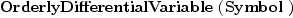(1)
Type: Type
fricas
-- here are the first 5 derivatives of w
-- the i-th derivative of w is printed as w subscript 5
[makeVariable('w,i)$odvar for i in 5..0 by -1]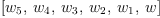(2) Type: List(OrderlyDifferentialVariable?(Symbol)) fricas -- these are now algebraic indeterminates, ranked in an orderly way -- in increasing order: sort %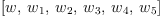(3) Type: List(OrderlyDifferentialVariable?(Symbol)) fricas -- we now make a general differential polynomial ring -- instead of ODVAR, one can also use SDVAR for sequential ordering dpol:=DSMP (FRAC INT, Symbol, odvar); Type: Type fricas -- instead of using makeVariable, it is easier to -- think of a differential variable w as a map, where -- w.n is n-th derivative of w as an algebraic indeterminate w := makeVariable('w)$dpol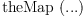(4)
Type: (NonNegativeInteger? -> DifferentialSparseMultivariatePolynomial?(Fraction(Integer),Symbol,OrderlyDifferentialVariable?(Symbol)))
fricas
-- create another one called z, which is higher in rank than w
-- since we are ordering by Symbol
z := makeVariable('z)\$dpol(5)
Type: (NonNegativeInteger? -> DifferentialSparseMultivariatePolynomial?(Fraction(Integer),Symbol,OrderlyDifferentialVariable?(Symbol)))
fricas
-- now define some differential polynomial
(f,b):dpol
Type: Void
fricas
f:=w.4::dpol - w.1 * w.1 * z.3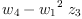(6)
Type: DifferentialSparseMultivariatePolynomial?(Fraction(Integer),Symbol,OrderlyDifferentialVariable?(Symbol))
fricas
b:=(z.1::dpol)^3 * (z.2)^2 - w.2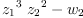(7)
Type: DifferentialSparseMultivariatePolynomial?(Fraction(Integer),Symbol,OrderlyDifferentialVariable?(Symbol))
fricas
-- compute the leading derivative appearing in b
lb:=leader b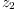(8)
Type: OrderlyDifferentialVariable?(Symbol)
fricas
-- the separant is the partial derivative of b with respect to its leader
sb:=separant b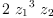(9)
Type: DifferentialSparseMultivariatePolynomial?(Fraction(Integer),Symbol,OrderlyDifferentialVariable?(Symbol))
fricas
-- of course you can differentiate these differential polynomials
-- and try to reduce f modulo the differential ideal generated by b
-- first eliminate z.3 using the derivative of b
bprime:= differentiate b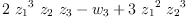(10)
Type: DifferentialSparseMultivariatePolynomial?(Fraction(Integer),Symbol,OrderlyDifferentialVariable?(Symbol))
fricas
-- find its leader
lbprime:= leader bprime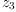(11)
Type: OrderlyDifferentialVariable?(Symbol)
fricas
-- differentiate f partially with respect to lbprime
pbf:=differentiate (f, lbprime)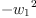(12)
Type: DifferentialSparseMultivariatePolynomial?(Fraction(Integer),Symbol,OrderlyDifferentialVariable?(Symbol))
fricas
-- to obtain the partial remainder of f with respect to b
ftilde:=sb * f- pbf * bprime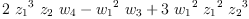(13)
Type: DifferentialSparseMultivariatePolynomial?(Fraction(Integer),Symbol,OrderlyDifferentialVariable?(Symbol))
fricas
-- note high powers of lb still appears in ftilde
-- the initial is the leading coefficient when b is written
-- as a univariate polynomial in its leader
ib:=initial b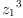(14)
Type: DifferentialSparseMultivariatePolynomial?(Fraction(Integer),Symbol,OrderlyDifferentialVariable?(Symbol))
fricas
-- compute the leading coefficient of ftilde
-- as a polynomial in its leader
lcef:=leadingCoefficient univariate(ftilde, lb)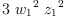(15)
Type: DifferentialSparseMultivariatePolynomial?(Fraction(Integer),Symbol,OrderlyDifferentialVariable?(Symbol))
fricas
-- now to continue eliminating the high powers of lb appearing in ftilde:
-- to obtain the remainder of f modulo b and its derivatives
f0:=ib * ftilde - lcef * b * lb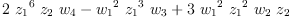(16)
Type: DifferentialSparseMultivariatePolynomial?(Fraction(Integer),Symbol,OrderlyDifferentialVariable?(Symbol))

 Subject:   Be Bold !! ( 14 subscribers )